# Polygamma function

In mathematics, the polygamma functions are the logarithmic derivatives of the gamma function. The polygamma function of order m is defined as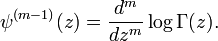$\psi^{(m-1)}(z) = \frac{d^m}{dz^m} \log \Gamma(z).$

The case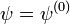$\psi = \psi^{(0)}\,\;$ is called the digamma function.

Taking the logarithm of Weierstrass's product for the gamma function, we can write the logarithm of the gamma function in the form of a series$\log \Gamma(z) = -\log z -\gamma z + \sum_{k=1}^\infty \left[ \frac{z}{k} - \log\left(1+\frac{z}{k}\right) \right].$

Differentiating termwise renders the logarithmic derivative of the gamma function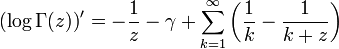$\left(\log \Gamma(z)\right)' = -\frac{1}{z} -\gamma + \sum_{k=1}^\infty \left(\frac{1}{k}-\frac{1}{k+z} \right)$

which is called the digamma function, denoted by$\psi(z)\,\!$. The expression for the digamma function is simpler than the series we started with, all logarithms notably absent. If z = n, a positive integer, all but finitely many terms in the series cancel and we are left with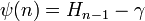$\psi(n) = H_{n-1}-\gamma\,\!$

where Hn is the harmonic number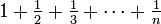$\textstyle 1 + \frac{1}{2} + \frac{1}{3} + \cdots + \frac{1}{n}$. As it generalizes harmonic numbers to non-integer indices, we could say that the digamma function is to harmonic numbers as the gamma function is to the factorials. Since (logf)' = f' / f, we can recover the ordinary derivative of the gamma function as Γ'(z) = Γ(z)ψ(z); the derivative at an integer is then Γ'(n) = (Hn − 1 − γ)(n − 1)! and in particular, Γ'(1) = − γ, providing a geometric interpretation of Euler's constant as the slope of the gamma function's graph at 1.

Continuing in a similar manner, rational series for the polygamma functions of higher order can be derived.

The polygamma functions are related to the Riemann zeta function: it can be shown that the polygamma function at an integer value is expressible in terms of the zeta function at integers. With the higher-order derivatives available, it becomes possible to calculate the Taylor series of the gamma function around any integer in terms of Euler's constant and the zeta function. Choosing 1 / Γ(z) instead of Γ(z) for convenience, since the former is an entire function and hence has an everywhere convergent Taylor series in the simple point z = 0, we can compute$\frac{1}{\Gamma(z)} = z + \gamma z^2 + \left(\frac{\gamma^2}{2} - \frac{\pi^2}{12}\right)z^3 + \cdots = \sum_{k=1}^\infty a_k z^k$

where a1 = 1, a2 = γ,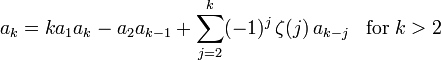$a_k = k a_1 a_k - a_2 a_{k-1} + \sum_{j=2}^k (-1)^j \, \zeta(j) \, a_{k-j} \;\;\; \mathrm{for}\; k > 2$

and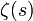$\zeta(s)\,\!$ is the Riemann zeta function.Some content on this page may previously have appeared on Citizendium.